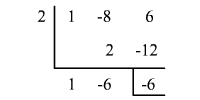# The Remainder Theorem

Generally when a polynomial is divided by a binomial there is a remainder.

Consider the polynomial function $f\left(x\right)={x}^{2}-8x+6$ . Divide the polynomial by the binomial $x-2$ .

We can do the division in either method.

### Method 1: Long Division

$\begin{array}{l}x-2\begin{array}{c}\hfill x-6\\ \hfill \overline{){x}^{2}-8x+6}\end{array}\\ \text{\hspace{0.17em}}\text{\hspace{0.17em}}\text{\hspace{0.17em}}\text{\hspace{0.17em}}\text{\hspace{0.17em}}\text{\hspace{0.17em}}\text{\hspace{0.17em}}\text{\hspace{0.17em}}\text{\hspace{0.17em}}\text{\hspace{0.17em}}\text{\hspace{0.17em}}\underset{_}{\text{\hspace{0.17em}}\text{\hspace{0.17em}}\text{\hspace{0.17em}}\text{\hspace{0.17em}}{x}^{2}-2x}\\ \text{\hspace{0.17em}}\text{\hspace{0.17em}}\text{\hspace{0.17em}}\text{\hspace{0.17em}}\text{\hspace{0.17em}}\text{\hspace{0.17em}}\text{\hspace{0.17em}}\text{\hspace{0.17em}}\text{\hspace{0.17em}}\text{\hspace{0.17em}}\text{\hspace{0.17em}}\text{\hspace{0.17em}}\text{\hspace{0.17em}}\text{\hspace{0.17em}}\text{\hspace{0.17em}}\text{\hspace{0.17em}}\text{\hspace{0.17em}}\text{\hspace{0.17em}}\text{\hspace{0.17em}}\text{\hspace{0.17em}}-6x+6\\ \text{\hspace{0.17em}}\text{\hspace{0.17em}}\text{\hspace{0.17em}}\text{\hspace{0.17em}}\text{\hspace{0.17em}}\text{\hspace{0.17em}}\text{\hspace{0.17em}}\text{\hspace{0.17em}}\text{\hspace{0.17em}}\text{\hspace{0.17em}}\text{\hspace{0.17em}}\text{\hspace{0.17em}}\text{\hspace{0.17em}}\text{\hspace{0.17em}}\text{\hspace{0.17em}}\text{\hspace{0.17em}}\text{\hspace{0.17em}}\text{\hspace{0.17em}}\text{\hspace{0.17em}}\underset{_}{\text{\hspace{0.17em}}-6x+12}\\ \text{\hspace{0.17em}}\text{\hspace{0.17em}}\text{\hspace{0.17em}}\text{\hspace{0.17em}}\text{\hspace{0.17em}}\text{\hspace{0.17em}}\text{\hspace{0.17em}}\text{\hspace{0.17em}}\text{\hspace{0.17em}}\text{\hspace{0.17em}}\text{\hspace{0.17em}}\text{\hspace{0.17em}}\text{\hspace{0.17em}}\text{\hspace{0.17em}}\text{\hspace{0.17em}}\text{\hspace{0.17em}}\text{\hspace{0.17em}}\text{\hspace{0.17em}}\text{\hspace{0.17em}}\text{\hspace{0.17em}}\text{\hspace{0.17em}}\text{\hspace{0.17em}}\text{\hspace{0.17em}}\text{\hspace{0.17em}}\text{\hspace{0.17em}}\text{\hspace{0.17em}}\text{\hspace{0.17em}}\text{\hspace{0.17em}}\text{\hspace{0.17em}}\text{\hspace{0.17em}}\text{\hspace{0.17em}}-6\text{\hspace{0.17em}}\text{\hspace{0.17em}}\text{\hspace{0.17em}}\end{array}$

The remainder is $-6$ .

### Method 2: Synthetic DivisionThe remainder is $-6$ .

Now compare the remainder of $-6$ to $f\left(2\right)$ .

$\begin{array}{l}f\left(2\right)=\left(2\right)-8\left(2\right)+6\\ =4-16+6\\ =-6\end{array}$

Notice that the value of $f\left(2\right)$ is the same as the remainder when the polynomial is divided by the binomial $x-2$ . This illustrates the Remainder Theorem.

If a polynomial $f\left(x\right)$ is divided by $x-a$ , the remainder is the constant $f\left(a\right)$ , and $f\left(x\right)=q\left(x\right)\cdot \left(x-a\right)+f\left(a\right)$ , where $q\left(x\right)$ is a polynomial with degree one less than the degree of $f\left(x\right)$ .

Synthetic division is a simpler process for dividing a polynomial by a binomial. When synthetic division is used to evaluate a function, it is called synthetic substitution.